# History of pi

23 de Jan de 2016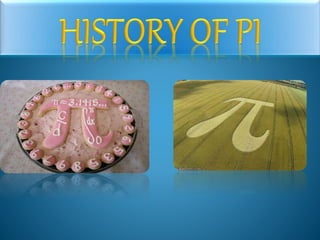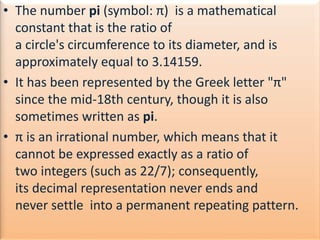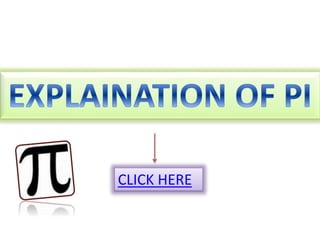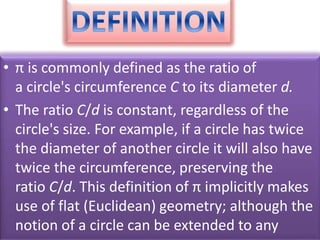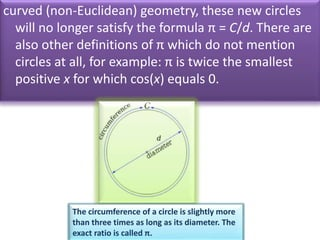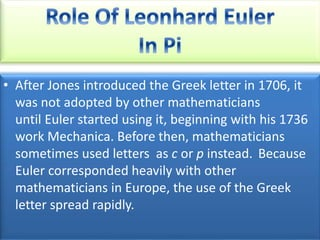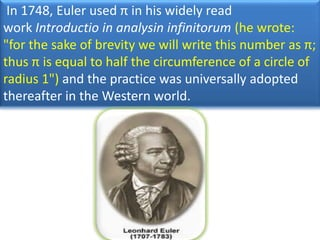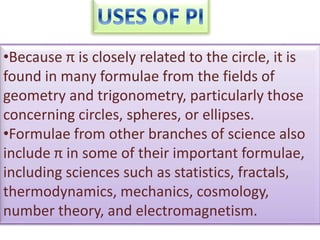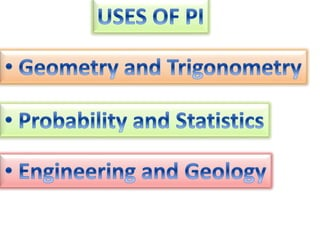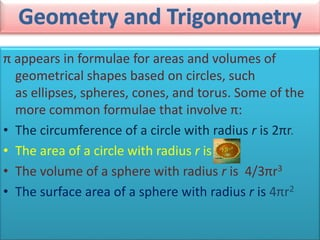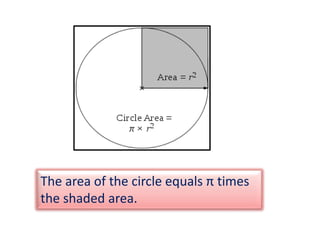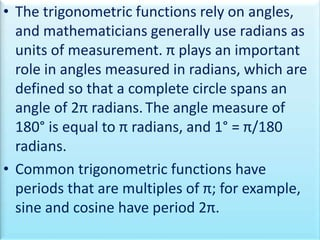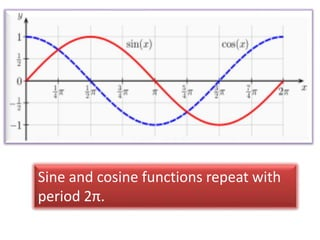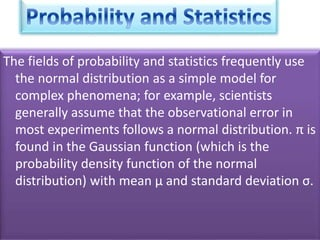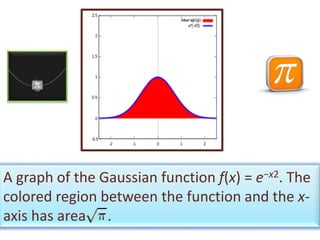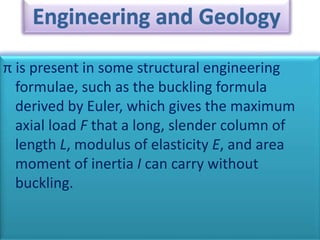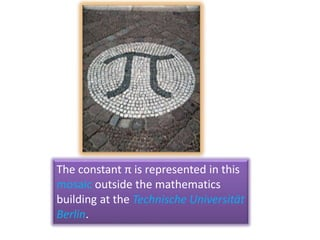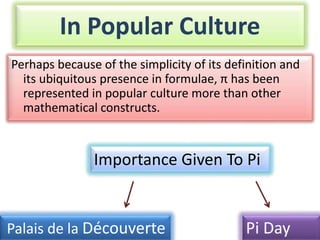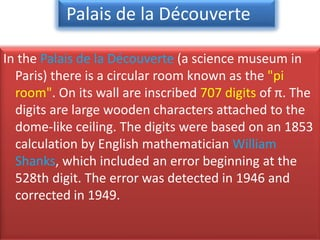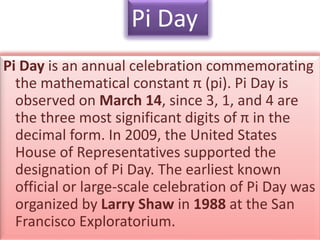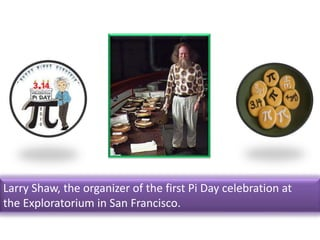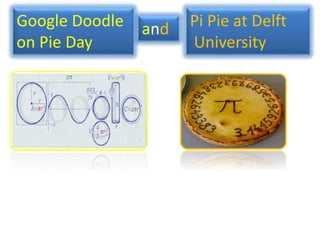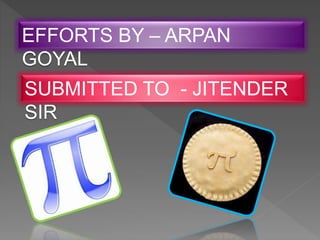1 de 27

### History of pi

• 2. • The number pi (symbol: π) is a mathematical constant that is the ratio of a circle's circumference to its diameter, and is approximately equal to 3.14159. • It has been represented by the Greek letter "π" since the mid-18th century, though it is also sometimes written as pi. • π is an irrational number, which means that it cannot be expressed exactly as a ratio of two integers (such as 22/7); consequently, its decimal representation never ends and never settle into a permanent repeating pattern.
• 4. • π is commonly defined as the ratio of a circle's circumference C to its diameter d. • The ratio C/d is constant, regardless of the circle's size. For example, if a circle has twice the diameter of another circle it will also have twice the circumference, preserving the ratio C/d. This definition of π implicitly makes use of flat (Euclidean) geometry; although the notion of a circle can be extended to any
• 5. curved (non-Euclidean) geometry, these new circles will no longer satisfy the formula π = C/d. There are also other definitions of π which do not mention circles at all, for example: π is twice the smallest positive x for which cos(x) equals 0. The circumference of a circle is slightly more than three times as long as its diameter. The exact ratio is called π.
• 6. • After Jones introduced the Greek letter in 1706, it was not adopted by other mathematicians until Euler started using it, beginning with his 1736 work Mechanica. Before then, mathematicians sometimes used letters as c or p instead. Because Euler corresponded heavily with other mathematicians in Europe, the use of the Greek letter spread rapidly.
• 7. In 1748, Euler used π in his widely read work Introductio in analysin infinitorum (he wrote: "for the sake of brevity we will write this number as π; thus π is equal to half the circumference of a circle of radius 1") and the practice was universally adopted thereafter in the Western world.
• 8. Leonhard Euler popularized the use of the Greek letter π in works he published in 1736 and 1748.
• 9. •Because π is closely related to the circle, it is found in many formulae from the fields of geometry and trigonometry, particularly those concerning circles, spheres, or ellipses. •Formulae from other branches of science also include π in some of their important formulae, including sciences such as statistics, fractals, thermodynamics, mechanics, cosmology, number theory, and electromagnetism.
• 11. π appears in formulae for areas and volumes of geometrical shapes based on circles, such as ellipses, spheres, cones, and torus. Some of the more common formulae that involve π: • The circumference of a circle with radius r is 2πr. • The area of a circle with radius r is • The volume of a sphere with radius r is 4/3πr3 • The surface area of a sphere with radius r is 4πr2
• 12. The area of the circle equals π times the shaded area.
• 13. • The trigonometric functions rely on angles, and mathematicians generally use radians as units of measurement. π plays an important role in angles measured in radians, which are defined so that a complete circle spans an angle of 2π radians.The angle measure of 180° is equal to π radians, and 1° = π/180 radians. • Common trigonometric functions have periods that are multiples of π; for example, sine and cosine have period 2π.
• 14. Sine and cosine functions repeat with period 2π.
• 15. The fields of probability and statistics frequently use the normal distribution as a simple model for complex phenomena; for example, scientists generally assume that the observational error in most experiments follows a normal distribution. π is found in the Gaussian function (which is the probability density function of the normal distribution) with mean μ and standard deviation σ.
• 16. A graph of the Gaussian function ƒ(x) = e−x2. The colored region between the function and the x- axis has area .
• 17. π is present in some structural engineering formulae, such as the buckling formula derived by Euler, which gives the maximum axial load F that a long, slender column of length L, modulus of elasticity E, and area moment of inertia I can carry without buckling.
• 18. The constant π is represented in this mosaic outside the mathematics building at the Technische Universität Berlin.
• 21. In Popular Culture Perhaps because of the simplicity of its definition and its ubiquitous presence in formulae, π has been represented in popular culture more than other mathematical constructs. Palais de la Découverte Pi Day Importance Given To Pi
• 22. In the Palais de la Découverte (a science museum in Paris) there is a circular room known as the "pi room". On its wall are inscribed 707 digits of π. The digits are large wooden characters attached to the dome-like ceiling. The digits were based on an 1853 calculation by English mathematician William Shanks, which included an error beginning at the 528th digit. The error was detected in 1946 and corrected in 1949. Palais de la Découverte
• 23. Pi Room
• 24. Pi Day is an annual celebration commemorating the mathematical constant π (pi). Pi Day is observed on March 14, since 3, 1, and 4 are the three most significant digits of π in the decimal form. In 2009, the United States House of Representatives supported the designation of Pi Day. The earliest known official or large-scale celebration of Pi Day was organized by Larry Shaw in 1988 at the San Francisco Exploratorium. Pi Day
• 25. Larry Shaw, the organizer of the first Pi Day celebration at the Exploratorium in San Francisco.
• 26. Google Doodle on Pie Day and Pi Pie at Delft University
• 27. EFFORTS BY – ARPAN GOYAL SUBMITTED TO - JITENDER SIR